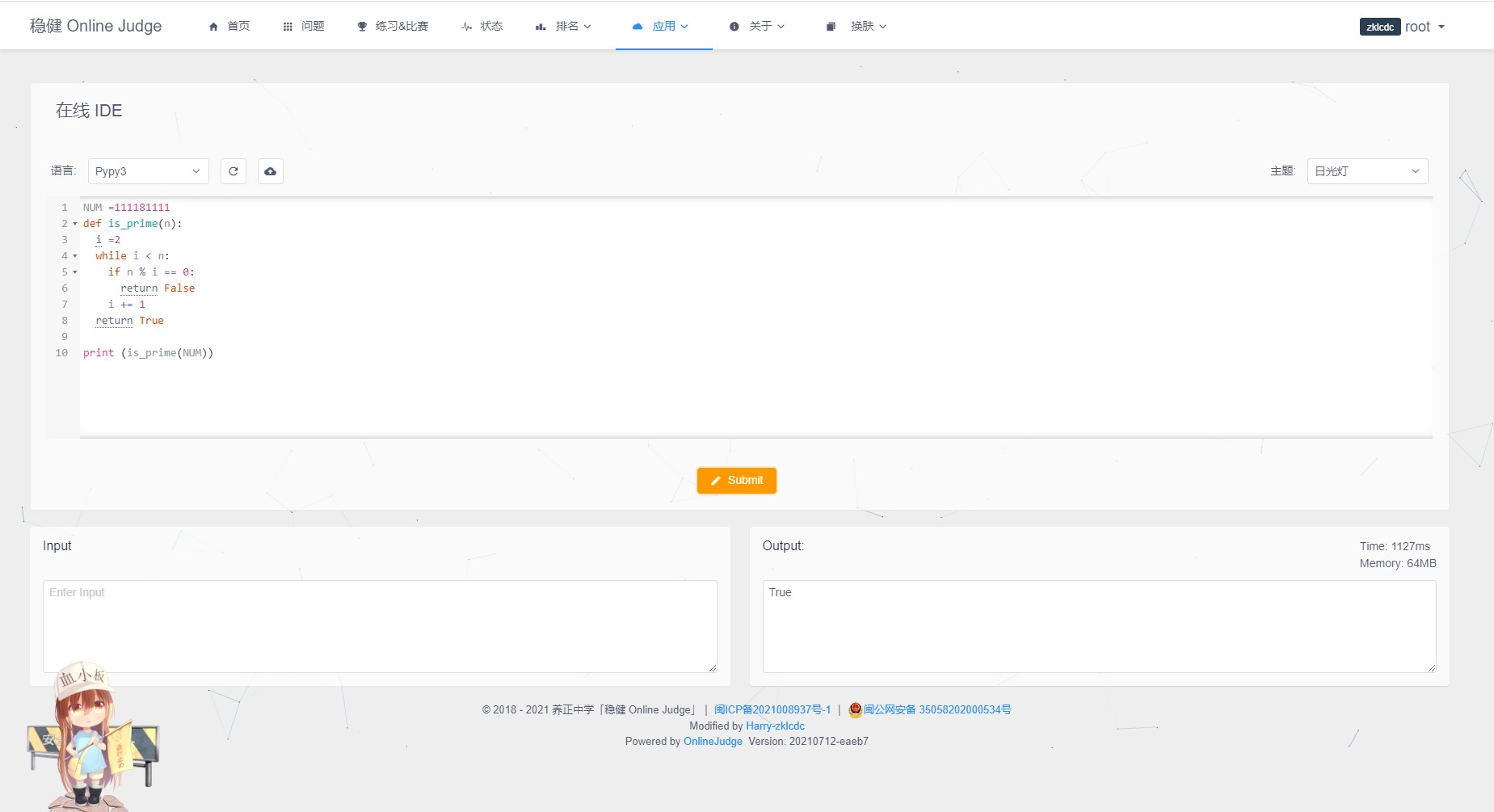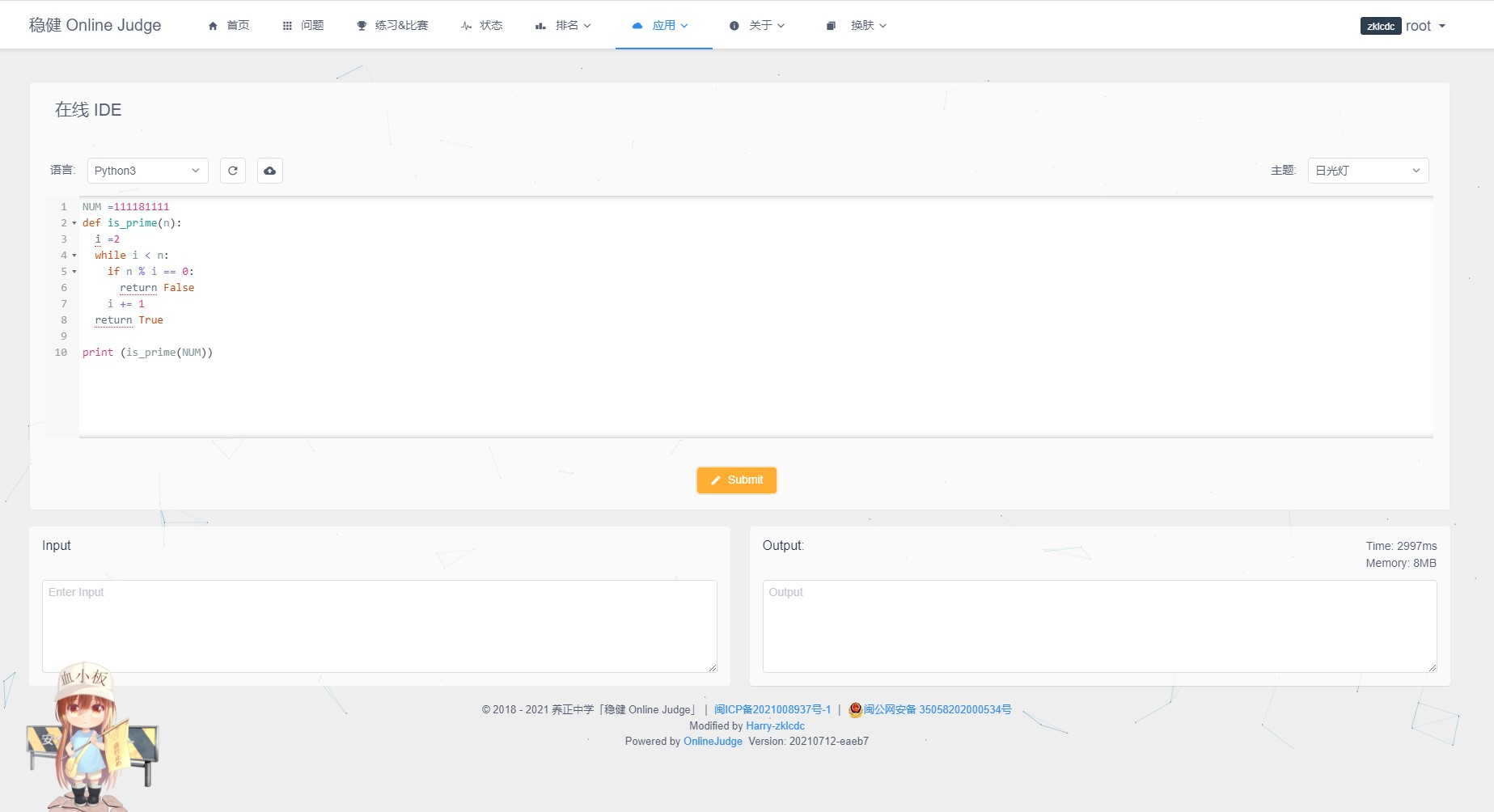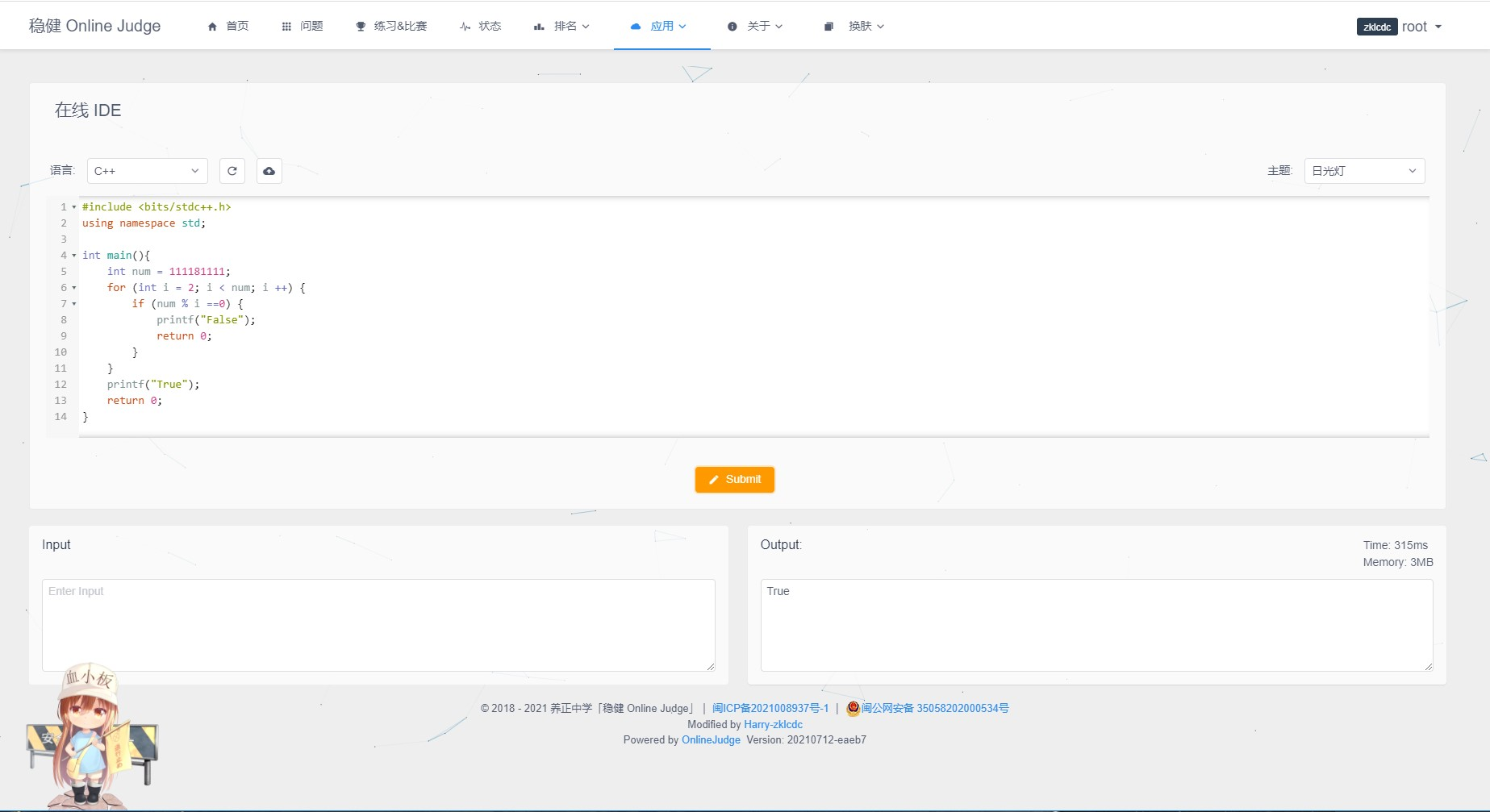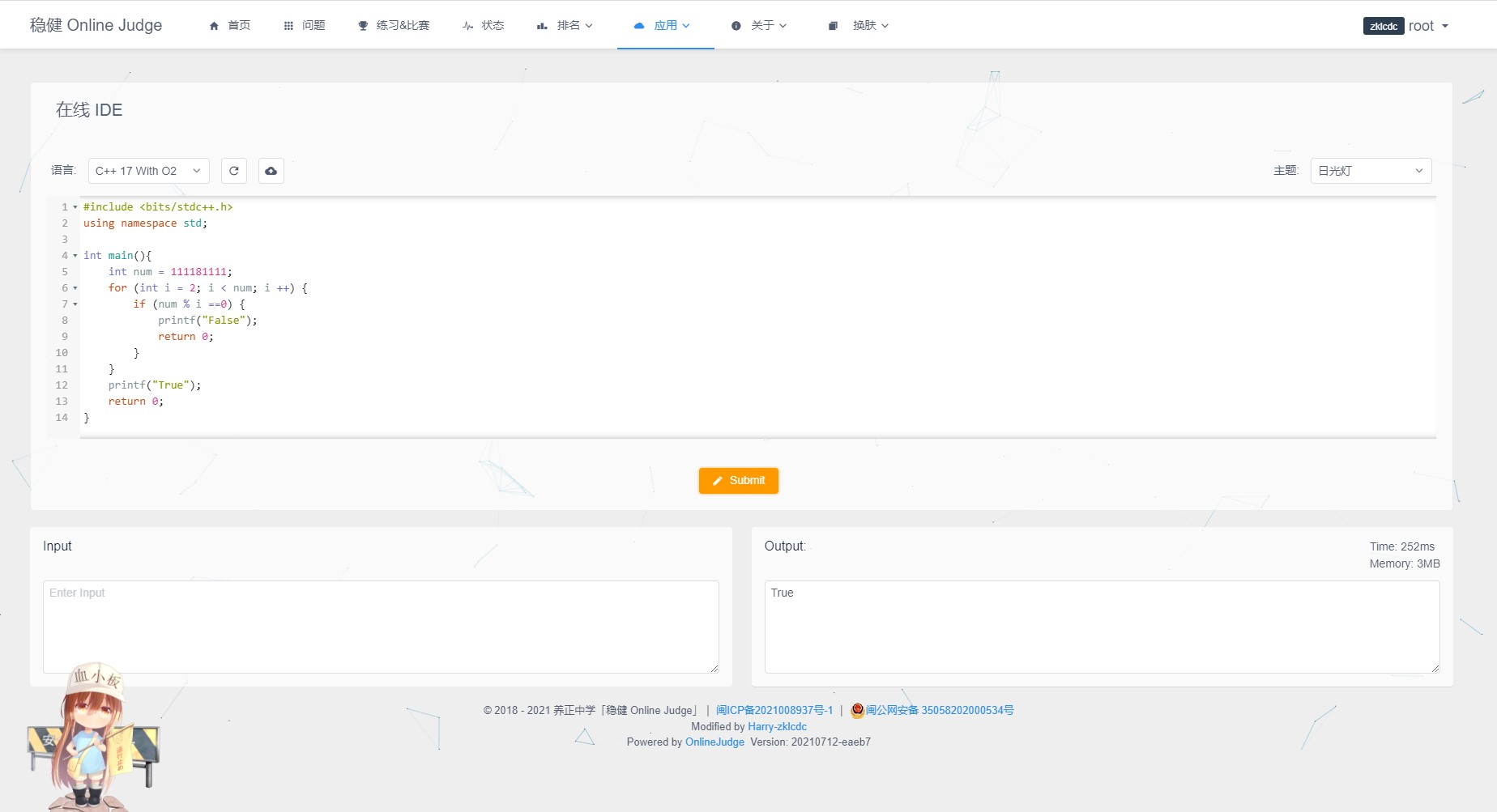“If you want your code to run faster, you should probably just use PyPy.” — Guido van Rossum (Creator of Python)## 什么是 Pypy？

Python代码为：

NUM =111181111
def is_prime(n):
i =2
while i < n:
if n % i == 0:
return False
i += 1
return True

print (is_prime(NUM))


C++代码为：

#include <bits/stdc++.h>
using namespace std;

int main(){
int num = 111181111;
for (int i = 2; i < num; i ++) {
if (num % i ==0) {
printf("False");
return 0;
}
}
printf("True");
return 0;
}


Pypy3的时间为：1127ms，如下图：Python3的时间为：TLE（超时），如下图：C++的时间为：315ms（不开启O2优化的情况下），开启O2优化能达到252ms（C++ yyds！），如下图：import time

start_time = time.time()
number = 0
for i in range(100000000):
number += i

print(f"Ellapsed time: {time.time() - start_time} s")


#include <bits/stdc++.h>
using namespace std;

int main(){
int num = 0;
double start_time = clock();
for (int i = 0; i < 100000000; i ++) {
num ++;
}
printf("Ellapsed time: %0.9f s", (double)(clock()- start_time) / CLOCKS_PER_SEC);
return 0;
}